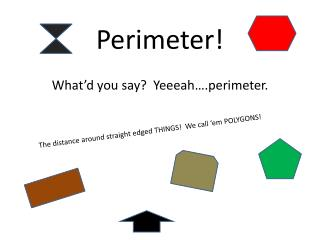DownloadDownload PresentationPerimeter!

# Perimeter!

Télécharger la présentation## Perimeter!

- - - - - - - - - - - - - - - - - - - - - - - - - - - E N D - - - - - - - - - - - - - - - - - - - - - - - - - - -
##### Presentation Transcript

1. Perimeter! The distance around straight edged THINGS! We call ‘em POLYGONS! What’d you say? Yeeeah….perimeter.

2. Key Terms Polygon ﻣﻀﻠﻊ Ex: a polygon is a shape that has straight edges. All the shapes on page 1 are polygons. Perimeter اﻟﻤﺤﻴﻂ Ex: The perimeter of a polygon is the distance around an object. Pentagon ﺧﻤﺎﺳﻲ اﻷﺿﻼع Ex: A pentagon is a five-sided polygon. Pentameans five and gonmeans shape. Property ﺧﺎﺻﻴﺔ Ex: What are the properties of an octagon? The properties of an octagon are that it is a eight-sided, straight-edged polygon. Octa means eight, and gon means shape.

3. Finding Perimeter 5 5 5 P = 20 5 5 5 1 1 5 5 5 1 1 7 10 7 P = 34 8 8 P = 43 8

4. Finding Perimeter with missing lengths 10 P = 40 10 10 5 5 5 5 ? 10 ? 5 5 ? P = 25 4 4 P = 17 4

5. How Do You Find The Perimeter? A) Find the product of the sides. Multiply B) Find the difference of the sides. Subtract C) Find the sum of the sides. Add D) Take a wild guess!